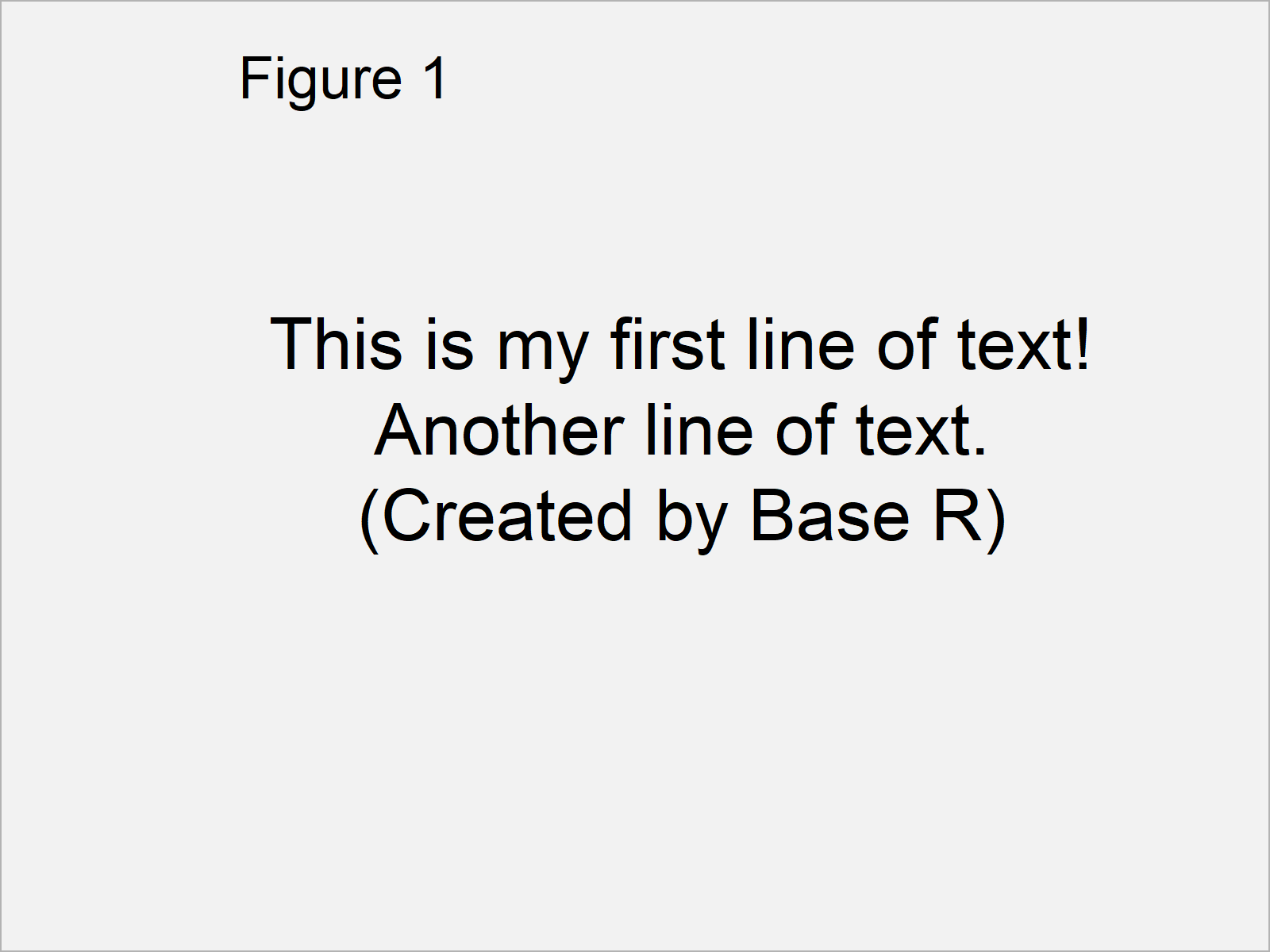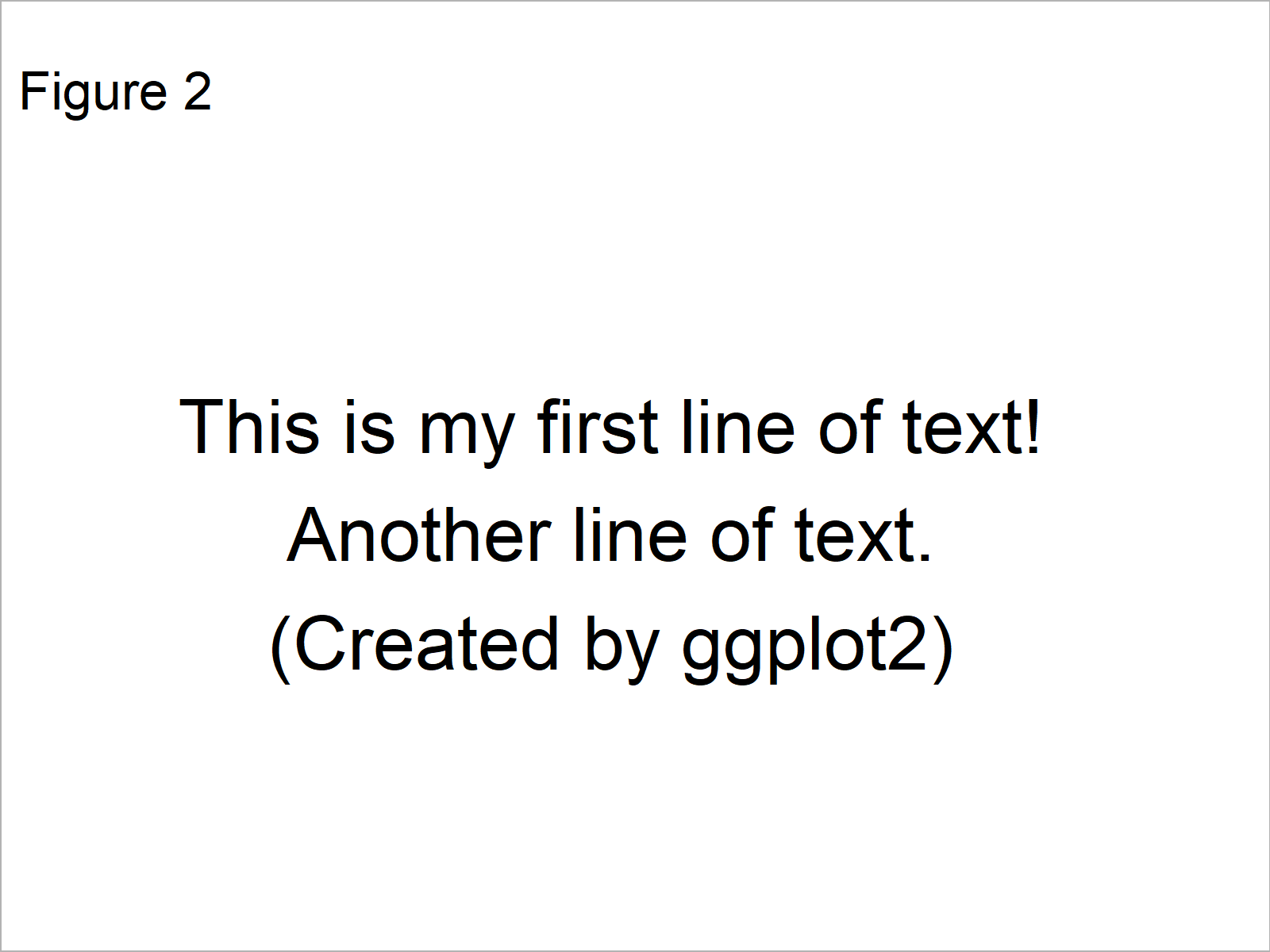# Plot Only Text in R (2 Examples)

In this tutorial, I’ll show how to create a graphic that contains only text in the R programming language.

The article contains the following information:

Here’s how to do it.

## Example 1: Show Only Text in Base R Plot

This section illustrates how to add text to an empty plot using the basic features of the R programming language.

In the first step, we have to remove all white space around our plot using the par function and the mar argument:

`par(mar = c(0, 0, 0, 0))        # Remove white space around plot`

Next, we can use a combination of the plot and text functions to create a plot containing only some text:

```plot(x = 0:1,                   # Create empty plot
y = 0:1,
ann = F,
bty = "n",
type = "n",
xaxt = "n",
yaxt = "n")
text(x = 0.5,                   # Add text to empty plot
y = 0.5,
"This is my first line of text!\nAnother line of text.\n(Created by Base R)",
cex = 1.8)```As shown in Figure 1, we have created a graph that consists only of text with the previous R programming code.

## Example 2: Show Only Text in ggplot2 Plot

As you have seen in Example 1, we can draw a plot with text only by using the basic installation of the R programming language.

However, an even easier solution is provided by the ggplot2 add-on package.

First, we have to install and load the ggplot2 package:

```install.packages("ggplot2")     # Install ggplot2 package

Next, we can use the ggplot and annotate functions to draw a plot with text only:

```ggplot() +                      # Draw ggplot2 plot with text only
annotate("text",
x = 1,
y = 1,
size = 8,
label = "This is my first line of text!\nAnother line of text.\n(Created by ggplot2)") +
theme_void()```As shown in Figure 2, the previous R programming syntax has plotted a ggplot2 plot with text only.

## Video, Further Resources & Summary

Do you need more explanations on the R programming codes of this page? Then you could have a look at the following video of my YouTube channel. In the video, I’m explaining the R programming code of this tutorial.

Please accept YouTube cookies to play this video. By accepting you will be accessing content from YouTube, a service provided by an external third party.If you accept this notice, your choice will be saved and the page will refresh.

Furthermore, you may read the other R programming posts on https://www.statisticsglobe.com/. You can find a selection of articles about topics such as ggplot2, text elements, and graphics in R below:

To summarize: You have learned in this tutorial how to display only text in a plot in R. If you have further questions, tell me about it in the comments. Furthermore, please subscribe to my email newsletter in order to get updates on new articles.

Subscribe to the Statistics Globe Newsletter